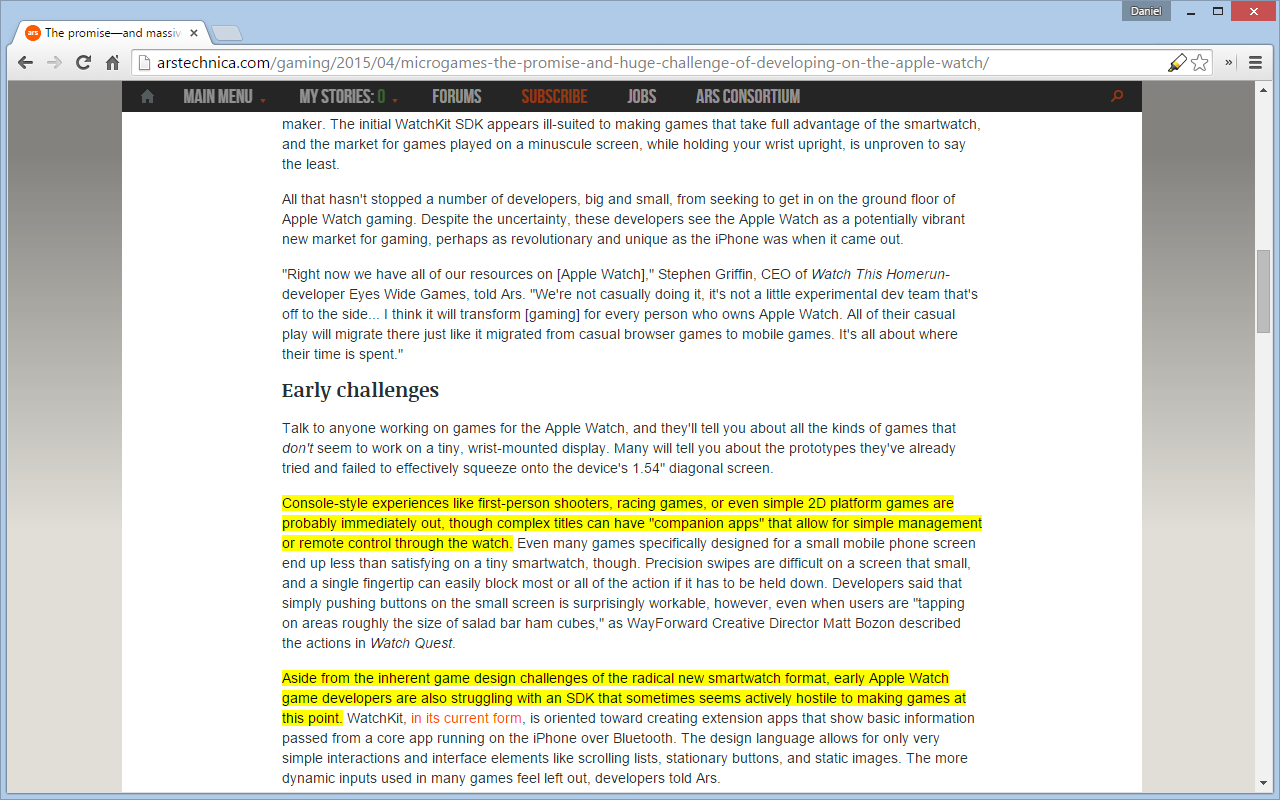Categories

## Factorization Machines with Theano

Update 11/4/2019: The github repo was renamed from PyFactorizationMachines to pyfms.

Update 4/20/2017: The library is now available on PyPI, the Python Package Index. It can be installed with pip.

$pip install pyfms A Factorization Machine (FM) is a predictive model that can be used for regression and classification (Rendle 2010). FMs efficiently incorporate pairwise interactions by using factorized parameters. PyFactorizationMachines is a Theano-based Python implementation of factorization machines. documentation, see documentation.md. For example usage, see example.py. Categories ## Conway’s Game of Life Here’s a quick-and-dirty implementation of Conway’s Game of Life. Cells can be selected/deselected by clicking and dragging your mouse. The interface and display were designed for use with a desktop/laptop computer, not a touchscreen mobile device. That is, cells can’t be selected by swiping (but tapping works). The default selected cells spell my first name, daniel. After selecting cells, click Start to begin the game of life. The source code is available here (use your browser’s view source). Categories ## Matrix Factorization with Theano Matrix factorization algorithms factorize a matrix D into two matrices P and Q, such that D ≈ PQ. By limiting the dimensionality of P and Q, PQ provides a low-rank approximation of D. While singular value decomposition (SVD) can also be used for this same task, the matrix factorization algorithms considered in this post accommodate missing data in matrix D, unlike SVD. For an overview of matrix factorization, I recommend Albert Au Yeung’s tutorial. That post describes matrix factorization, motivates the problem with a ratings prediction task, derives the gradients used by stochastic gradient descent, and implements the algorithm in Python. For exploratory work, it would be great if the algorithm could be implemented in such a way that the gradients could be automatically derived. With such an approach, gradients would not have to be re-derived when e.g., a change is made to the loss function (either the error term and/or the regularization term). In general, automatically derived gradients for machine learning problems facilitate increased exploration of ideas by removing a time-consuming step. Theano is a Python library that allows users to specify their problem symbolically using NumPy-based syntax. The expressions are compiled to run efficiently on actual data. Theano’s webpage provides documentation and a tutorial. The following code includes a Theano-based implementation of matrix factorization using batch gradient descent. The parameters are similar to those in the quuxlabs matrix factorization implementation. D is a second-order masked numpy.ndarray (e.g., a ratings matrix, where the mask indicates missing ratings), and P and Q are the initial matrix factors. The elements of P and Q are the parameters of the model, which are initialized by the function’s caller. The rank of the factorization is specified by the dimensions of P and Q. For a rank-k factorization, P must be$m \times k$ and Q must be$k \times n$ (where D is an$m \times n$ matrix). Additional parameters specify the number of iterations, the learning rate, and the regularization importance. The code doesn’t contain any derived gradients. It specifies the loss function and the parameters that the loss function will be minimized with respect to. Theano figures out the rest! This file contains bidirectional Unicode text that may be interpreted or compiled differently than what appears below. To review, open the file in an editor that reveals hidden Unicode characters. Learn more about bidirectional Unicode characters  import numpy as np import numpy.ma as ma import theano from theano import tensor as T floatX = theano.config.floatX def getmask(D): return ma.getmaskarray(D) if ma.isMA(D) else np.zeros(D.shape, dtype=bool) def matrix_factorization_bgd( D, P, Q, steps=5000, alpha=0.0002, beta=0.02): P = theano.shared(P.astype(floatX)) Q = theano.shared(Q.astype(floatX)) X = T.matrix() error = T.sum(T.sqr(~getmask(D) * (P.dot(Q) – X))) regularization = (beta/2.0) * (T.sum(T.sqr(P)) + T.sum(T.sqr(Q))) cost = error + regularization gp, gq = T.grad(cost=cost, wrt=[P, Q]) train = theano.function(inputs=[X], updates=[(P, P – gp * alpha), (Q, Q – gq * alpha)]) for _ in xrange(steps): train(D) return P.get_value(), Q.get_value() Categories ## Article Highlighter Auto Highlight is a Chrome extension that automatically highlights the important content on article pages. Here’s a link to the extension: https://chrome.google.com/webstore/detail/highlight/dnkdpcbijfnmekbkchfjapfneigjomhh The source code is on GitHub: https://github.com/dstein64/highlight After installing the extension, a highlighter icon appears in the location bar. Clicking that icon highlights important content on the page.Categories ## Anchor Graph Hashing in Python Update 11/7/2019: The github repo was renamed from PyAnchorGraphHasher to aghasher. The library is now available on PyPI, the Python Package Index. It can be installed with pip. $ pip install aghasher

I was recently collaborating on a project that relied on hashing (where I’m referring to “hashing” the same way it’s used in locality sensitive hashing, as opposed to its more conventional usage). One of my contributions was an implementation of Anchor Graph Hashing (AGH)  in Python. The code was integrated into the project, but I’ve uploaded the AGH module to its own separate GitHub repository.

https://github.com/dstein64/PyAnchorGraphHasher

 Liu, Wei, Jun Wang, Sanjiv Kumar, and Shih-Fu Chang. 2011. “Hashing with Graphs.” In Proceedings of the 28th International Conference on Machine Learning (ICML-11), edited by Lise Getoor and Tobias Scheffer, 1–8. ICML ’11. New York, NY, USA: ACM.